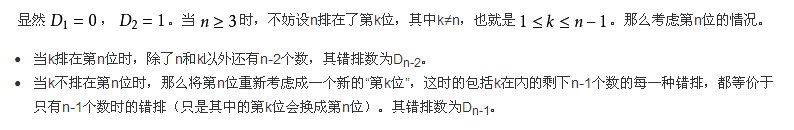# BZOJ4517: [Sdoi2016]排列计数(组合数+错位排列)

Time Limit: 60 Sec  Memory Limit: 128 MB
Submit: 1626  Solved: 994
[Submit][Status][Discuss]

## Description

1 ~ n 这 n 个数在序列中各出现了一次

## Input

T=500000，n≤1000000，m≤1000000

5
1 0
1 1
5 2
100 50
10000 5000

0
1
20
578028887
60695423

## Source#include<cstdio>
#define int long long
using namespace std;
const int mod=1e9+7;
const int MAXN=1e6+10;
{
char c=getchar();int x=0,f=1;
while(c<'0'||c>'9'){if(c=='-')f=-1;c=getchar();}
while(c>='0'&&c<='9'){x=x*10+c-'0';c=getchar();}
return x*f;
}
int fac[MAXN],D[MAXN],inv[MAXN];
void Pre()
{
fac=fac=inv=inv=D=D=1;
for(int i=2;i<=1000001;i++) fac[i]=(i*fac[i-1])%mod;
for(int i=2;i<=1000001;i++) inv[i]=(mod-mod/i)*inv[mod%i]%mod;
for(int i=2;i<=1000001;i++) inv[i]=(inv[i]*inv[i-1])%mod;
for(int i=3;i<=1000001;i++) D[i]=((i-1)*(D[i-1]+D[i-2]))%mod;
}
int Query(int N,int M)
{
return fac[N] %mod * inv[ N-M  ] %mod * inv[ M ] %mod * D[N-M] %mod;
}
main()
{
Pre();
while(T--)
{
}